Home

# Sets of large values of correlation functions for polynomial cubic configurations

## Abstract

We prove that for any set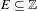$E\subseteq \mathbb{Z}$ with upper Banach density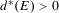$d^{\ast }(E)>0$ , the set ‘of cubic configurations’ in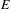$E$ is large in the following sense: for any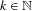$k\in \mathbb{N}$ and any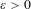$\unicode[STIX]{x1D700}>0$ , the set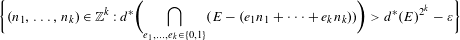$$\begin{eqnarray}\displaystyle \biggl\{(n_{1},\ldots ,n_{k})\in \mathbb{Z}^{k}:d^{\ast }\biggl(\mathop{\bigcap }_{e_{1},\ldots ,e_{k}\in \{0,1\}}(E-(e_{1}n_{1}+\cdots +e_{k}n_{k}))\biggr)>d^{\ast }(E)^{2^{k}}-\unicode[STIX]{x1D700}\biggr\} & & \displaystyle \nonumber\end{eqnarray}$$
is an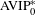$\text{AVIP}_{0}^{\ast }$ -set. We then generalize this result to the case of ‘polynomial cubic configurations’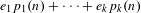$e_{1}p_{1}(n)+\cdots +e_{k}p_{k}(n)$ , where the polynomials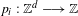$p_{i}:\mathbb{Z}^{d}\longrightarrow \mathbb{Z}$ are assumed to be sufficiently algebraically independent.

Hide All

# Sets of large values of correlation functions for polynomial cubic configurations

## Metrics

### Full text viewsFull text views reflects the number of PDF downloads, PDFs sent to Google Drive, Dropbox and Kindle and HTML full text views.

Total number of HTML views: 0
Total number of PDF views: 0 *Loading metrics...

### Abstract viewsAbstract views reflect the number of visits to the article landing page.

Total abstract views: 0 *Loading metrics...

* Views captured on Cambridge Core between <date>. This data will be updated every 24 hours.

Usage data cannot currently be displayed.